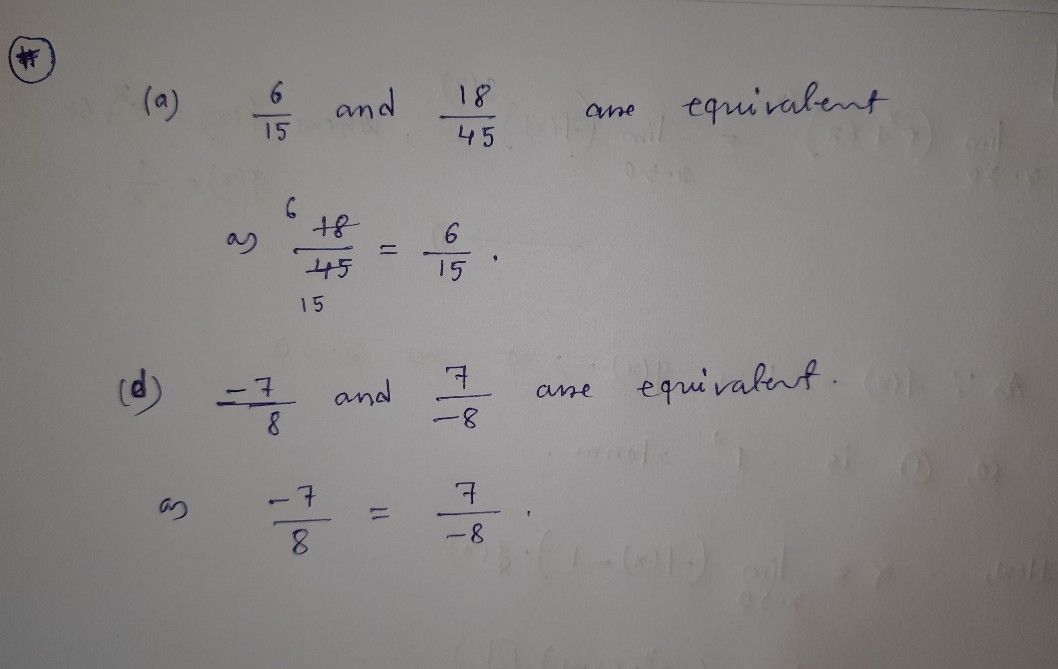Symbol
ProblemE Which of the following pairs of rational numbers are equivalent? $\left(2\right)$ $\dfrac {6} {15}$ and $\dfrac {18} {45}$ $\left($ (b) $\dfrac {12} {9}$ and $\dfrac {5} {3}$ $\left(c\right)$ $\dfrac {6} {5}$ and $\dfrac {4} {3}$ $\left($ (d) $\dfrac {-7} {8}$ and $\dfrac {7} {-8}$ $△$ $△$